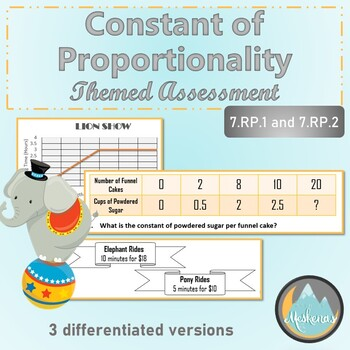# Constant of Proportionality Assessment - THREE versions 7.RP.1 and 7.RP.2Subject
Resource Type
File Type

PDF

(2 MB|20 pages)
Product Rating
Standards
• Product Description
• StandardsNEW

This Project-Based Assessment fully covers the 7.RP.1 and 7.RP.2 standards. It is designed to make differentiation a breeze! There are THREE versions of the same assessment (modified, traditional, and advanced), ranging in difficulty level, in order to accurately assess a range of students. Answer sheets and answer keys are included for all three versions of the assessment.

Students will be challenged to determine proportionality/find the unit rate with:

-Tables

-Graphs

-Verbal Descriptions

Students will be challenged to interpret the meaning of ordered pairs and predict future values in a proportional relationship.

Explain what a point (𝘹, 𝘺) on the graph of a proportional relationship means in terms of the situation, with special attention to the points (0, 0) and (1, 𝘳) where 𝘳 is the unit rate.
Represent proportional relationships by equations. For example, if total cost 𝘵 is proportional to the number 𝘯 of items purchased at a constant price 𝘱, the relationship between the total cost and the number of items can be expressed as 𝘵 = 𝘱𝘯.
Identify the constant of proportionality (unit rate) in tables, graphs, equations, diagrams, and verbal descriptions of proportional relationships.
Decide whether two quantities are in a proportional relationship, e.g., by testing for equivalent ratios in a table or graphing on a coordinate plane and observing whether the graph is a straight line through the origin.
Recognize and represent proportional relationships between quantities.
Total Pages
20 pages
Included
Teaching Duration
45 minutes
Report this Resource to TpT
Reported resources will be reviewed by our team. Report this resource to let us know if this resource violates TpT’s content guidelines.